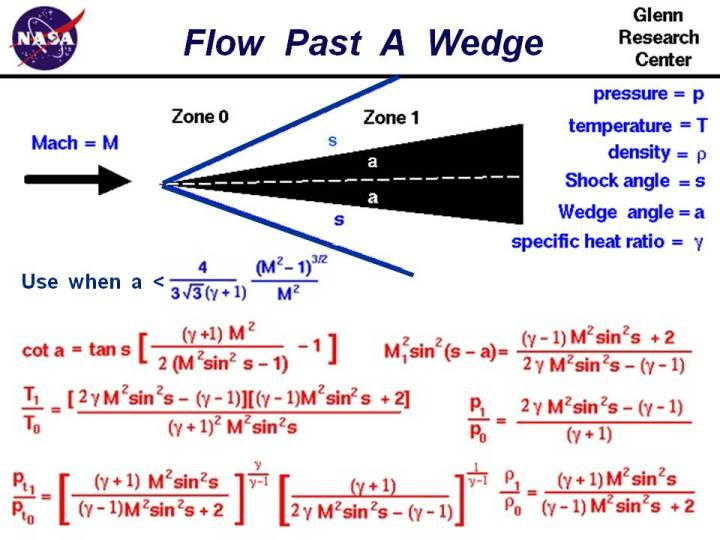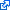+ Text Only Site
+ Non-Flash Version
+ Contact GlennAs an object moves through a gas, the gas molecules are deflected around the object. If the speed of the object is much less than the speed of sound of the gas, the density of the gas remains constant and the flow of gas can be described by conserving momentum, and energy. As the speed of the object approaches the speed of sound, we must consider compressibility effects on the gas. The density of the gas varies locally as the gas is compressed by the object. For compressible flows with little or small flow turning, the flow process is reversible and the entropy is constant. The change in flow properties are then given by the isentropic relations (isentropic means "constant entropy"). But when an object moves faster than the speed of sound, and there is an abrupt decrease in the flow area, shock waves are generated in the flow. Shock waves are very small regions in the gas where the gas properties change by a large amount. Across a shock wave, the static pressure, temperature, and gas density increases almost instantaneously. The changes in the flow properties are irreversible and the entropy of the entire system increases. Because a shock wave does no work, and there is no heat addition, the total enthalpy and the total temperature are constant. But because the flow is non-isentropic, the total pressure downstream of the shock is always less than the total pressure upstream of the shock. There is a loss of total pressure associated with a shock wave. On this page, we consider the supersonic flow of air past a two-dimensional wedge. If the Mach number is high enough and the wedge angle is small enough, an oblique shock wave is generated by the wedge, with the origin of the shock attached to the sharp leading edge of the wedge. If we think of the oblique shock as a normal shock inclined to the flow at some shock angle s, then the normal shock relations can be applied across the shock in a direction perpendicular to the shock, and the flow component parallel to the shock remains unchanged. The resulting Mach number and speed of the flow decrease across the shock wave. For the Mach number change across an oblique shock there are two possible solutions; one supersonic and one subsonic. In nature, the supersonic ("weak shock") solution occurs most often. However, under some conditions the "strong shock", subsonic solution is possible. For a given upstream Mach number, there is a maximum wedge angle for which the shock remains attached to the leading edge. For wedge angles greater than the maximum, a detached normal shock occurs. The conditions for an attached shock is shown on the slide. a < (4 * (M^2 -1)^3/2) /(M^2 * 3 * sqrt(3) * (gamma+1)) where M is the free stream Mach number, a is the wedge angle measured in radians, and gamma is the ratio of specific heats for the gas (=1.4 for air at sea -level standard temperature and pressure). On the slide we have listed the equations which describe the change in flow variables for flow past a two dimensional shock generated by a wedge of angle a. The equations presented here were derived by considering the conservation of mass, momentum, and energy for a compressible gas while ignoring viscous effects. The equations have been further specialized for a two-dimensional flow (not three dimensional axisymmetric) without heat addition. The equations only apply for those combinations of free stream Mach number and wedge angle for which an attached oblique shock occurs. If the Mach number is too low, or the wedge angle too high, the normal shock equations should be used. For the problem given on the slide, a supersonic flow at Mach number M approaches a wedge of angle a. A shock wave is generated which is inclined at angle s. Then: cot(a) = tan(s) * [{((gam+1) * M^2)/(2 * M^2 * sin^2(s) - 1)} - 1] where tan is the trigonometric tangent function, cot is the co-tangent function: cot(a) = tan(90 degrees - a) and sin^2 is the square of the sine. Gam is the ratio of specific heats. Across the shock wave the Mach number decreases to a value specified as M1: M1^2 * sin^2(s -a) = [(gam-1)M^2 sin^2(s) + 2] / [2 * gam * M^2 * sin^2(s) - (gam - 1)] The total temperature across the shock is constant, but the static temperature T increases in zone 1 to become: T1 / T0 = [2 * gam * M^2 * sin^2(s) - (gam - 1)] * [(gam -1) * M^2 * sin^2(s) + 2] / [(gam + 1)^2 * M^2 * sin^2(s)] The total pressure pt decreases according to: pt1 / pt0 = {[(gam + 1) * M^2 * sin^2(s)]/[(gam-1)*M^2 * sin^2(s) + 2]}^[gam/((gam-1)] * {(gam+1)/[2 * gam * M^2 * sin^2(s)-(gam-1)]}^[1/(gam-1)] The static pressure p increases to: p1 / p0 = [2 * gam * M^2 * sin^2(s)-(gam -1)] / (gam + 1) And the density r changes by: r1 / r0 = [(gam + 1) * M^2 * sin^2(s)] / [(gam -1) * M^2 * sin^2(s) + 2] The right hand side of all these equations depend only on the free stream Mach number and the shock angle. The shock angle depends in a complex way on the free stream Mach number and the wedge angle. So knowing the Mach number and the wedge angle, we can determine all the conditions associated with the oblique shock. The equations describing oblique shocks were published in NACA report (NACA-1135) in 1951. Here's a Java program based on the oblique shock equations. You can use this simulator to study the flow past a wedge. Due to IT security concerns, many users are currently experiencing problems running NASA Glenn educational applets. The applets are slowly being updated, but it is a lengthy process. If you are familiar with Java Runtime Environments (JRE), you may want to try downloading the applet and running it on an Integrated Development Environment (IDE) such as Netbeans or Eclipse. The following are tutorials for running Java applets on either IDE: NetbeansEclipseThis page shows an interactive Java applet for supersonic flow past a wedge. The applet shows the shock wave generated by the wedge and the value of the flow variables downstream of the shock. Input to the program can be made using the sliders, or input boxes at the upper right. To change the value of an input variable, simply move the slider. Or click on the input box, select and replace the old value, and hit Enter to send the new value to the program. Output from the program is displayed in output boxes at the lower right. The flow variables are presented as ratios to free stream values. The graphic at the left shows the wedge (in red) and the shock wave generated by the wedge as a line. The line is colored blue for an oblique shock and magenta when the shock is a normal shock. The black lines show the streamlines of the flow past the wedge. Notice that downstream (to the right) of the shock wave, the lines are closer together than upstream. This indicates an increase in the density of the flow. There is more complete shock simulation program that is also avaliable at this web site. It solves for both flow past a wedge and flow past a cone. If you are an experienced user of the simulator, you can use a sleek version of the program which loads faster on your computer and does not include the user's manual. The ShockModeler program models the intersection and reflection of multiple oblique shock waves generated by multiple wedges. You can also download your own copy of the program to run off-line by clicking on this button: Activities: Guided ToursCompressible Aerodynamics:Shock Waves and Expansions:Single Shock Wave Simulator:Multiple Shock Wave Simulator:Sources of Drag:Inlet:Navigation ..Beginner's Guide Home Page+ Inspector General Hotline + Equal Employment Opportunity Data Posted Pursuant to the No Fear Act + Budgets, Strategic Plans and Accountability Reports + Freedom of Information Act + The President's Management Agenda + NASA Privacy Statement, Disclaimer, and Accessibility CertificationEditor: Nancy Hall NASA Official: Nancy Hall Last Updated: May 13 2021 + Contact Glenn